Courses

# RD Sharma Solutions -Ex-18.2, Basic Geometrical Tools, Class 6, Maths Class 6 Notes | EduRev

## RD Sharma Solutions for Class 6 Mathematics

Created by: Abhishek Kapoor

## Class 6 : RD Sharma Solutions -Ex-18.2, Basic Geometrical Tools, Class 6, Maths Class 6 Notes | EduRev

The document RD Sharma Solutions -Ex-18.2, Basic Geometrical Tools, Class 6, Maths Class 6 Notes | EduRev is a part of the Class 6 Course RD Sharma Solutions for Class 6 Mathematics.
All you need of Class 6 at this link: Class 6

Q. 1.) Mark the two points, A and B on a piece of paper and join them. Measure this length. For each of the following draw a line segment CD that is:

(i) Equal to the segment AB

(ii) Twice AB

(iii) three times AB

(iv) Half AB

(v) collinear with AB and is equal to it.

Mark two points, A and B on a piece of paper and join them as follows: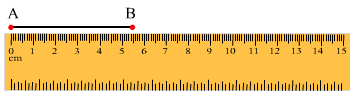To measure the length of AB, place the ruler with its edge along AB, such that the zero mark of the cm side of the ruler coincides with point A, as shown in the figure. Now, read the mark on the ruler, which corresponds to the point B. The reading on the ruler at point B is the length of the line segment AB. Here, AB = 5.6 cm

(i) To draw the line segment CD equal to AB, take a divider and open it, such that the end-point of one of its arms is at A and the end-point of the second arm is at B, as shown in the figure. Then, lift the divider and without disturbing its opening, place the end-points of both hands on the paper, where we have to draw CD.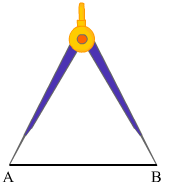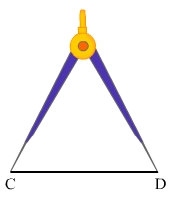(ii) To draw the line segment twice AB, draw a line / and take a point C on it. Now, take a divider and open it such that the end points of both its arms are at A and B. Then, lift the divider and without disturbing its opening, place one end-point at C and the other end-point on the line 1, as shown in the figure. Lift the divider and place one end-point at E and the other end-point on the line 1, opposite C. Name this point D.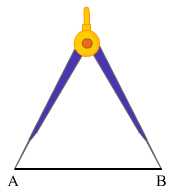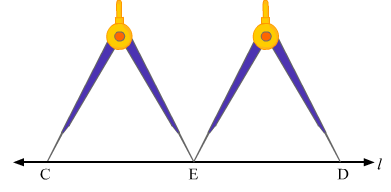(iii) To draw the line segment three times A, we draw a line / and take a point C on it. Now take a divider and open it, such that the end-points of both its arms are at A and B.

Then, we lift the divider and place one end-point at C and the other end-point on the line 1, as shown in the figure.

Let this point be E.

Again, lift the divider and place one end-pint at E and the other end-point on the line 1, opposite to C. Let this point be F.

Again, lift the divider and place one end-point at F and the other end-point on the line 1, opposite to C. Name this point D.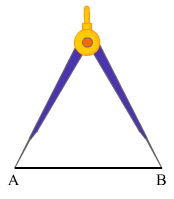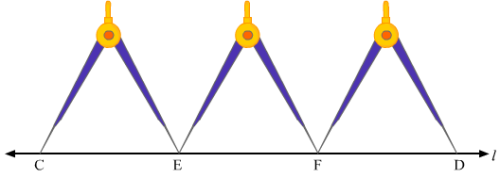(iv) To draw the line segment that is half AB, we draw a line / and take a point C on it. Now, using a ruler, we measure the line segment AB and here, AB = 5.6 cm

Half of AB=5.62=2.8 cm

Now, we take a divider and open it so much that its end of one hand is at 0 and end of the another hand is at 2.8 cm

Then, we lift the divider and place one end at C and the other end on the line l at point D.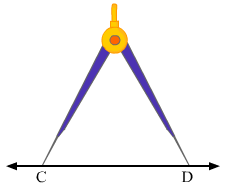(v) To draw a line segment CD collinear with AB and equal to AB, we take a ruler along AB and draw the line extended to AB, as shown in the figure. We take a divider and open it such that the end-points of both its arms are at A and B. Then, we lift the divider and place the end-points of both its hands on the extended line of AB and mark them as C and D.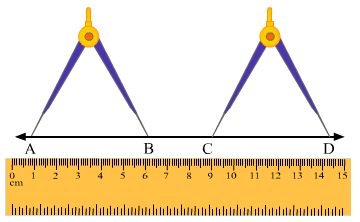Q. 2.) The end – point P of a line – segment PQ is against 4 cm mark and the end – point Q is against the mark indicating 14.8 cm on a ruler. What is the length of the segment PQ?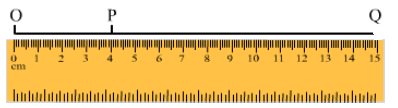Extend the line segment QP towards point zero of the ruler and take a point 0 on the extended line QP corresponding to point zero on the ruler.

From the figure, we can say:

OP = 4 cm and OQ = 14.8 cm

Now, PQ = OQ – OP

= (14.8 - 4) cm

= 10.8 cm

Q. 3.) Draw a line segment CD. Produce it to CE such that CE= 3 CD

We draw a line l and take two points C and D on it.

Take a divider and open it such that its end of both arms is at C and D.

Then, we lift the divider and place its one end at D and other end on the line l opposite to C as shown in the figure.

Let this point be A.

Lift the divider again and place its one end at A and other end on the line 1 opposite to C.

Name this point as E.

Here CD = DE = AE

Therefore, CE = CD + DE + AE

= CD + CD + CD (As, CD ± DE = AE)

or, CE = 3CD

Q. 4.) If AB = 7.5 cm and CD = 2.5 cm, construct a segment whose length is equal to

(i) AB – CD

(ii) 2 AB

(iii) 3 CD

(iv) AB + CD

(v) 2 AB + 3 CD

Given:

AB= 7.5 cm and CD = 2.5 cm

Draw AB and CD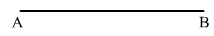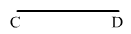(i) Draw a line l and take a point E on it.

Now, take a divider and open it such that ends of both the arms are at A and B. Then, we lift the divider and place its one end at E and other end (F) and on the line l as shown in figure. Now, reset the divider in such a way that the end of its one hand is at C and the end of the other hand is at D. Then, we lift the divider and place its one end at E and other end (G) on the line l as shown in the figure. FG is required line segment, whose length is equal to (AB – CD)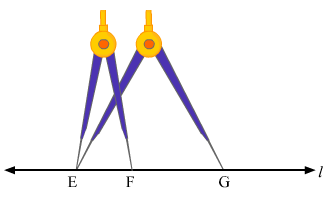(ii) Draw a line l and take a point E on it. Now a take a divider and open it such that the ends of both its arms are at A and B.

Then, we lift the divider and place its one end at E and other end (say F) on the line l as shown in the figure.

Again, lift the divider and place its one end F and other end on the line l, opposite to E.

Let this point be G.

EG is required line segment, whose length is equal to 2 AB.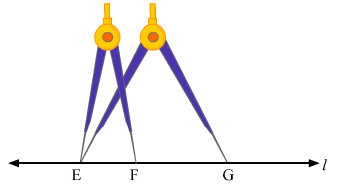(iii) Draw a line l and take a point E on it. Now take a divider and open it such that the ends of both its arms are at C and D.

Then, we lift the divider and place its end at E on it and other end (F) on the line l, as shown in the figure.

Again, we lift the divider end (G) on the l opposite to C.

Again, lift the divider end (G) on the line l opposite to C.

Again, lift the divider and place its one end at G and another end (H) on the line l, opposite to E. EH is required line segment, whose length is equal to 3 CD.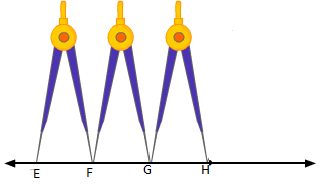(iv) We draw a line l and take a point E on it. Now, take a divider and open it such that the ends of both its arms are A and B. The, we lift the divider end (F) on the line l, as shown in the figure.

Now, reset the divider in such a way that the end of its one hand is at C and the end of the other hand is at D.

Then, we lift the divider and place its one end at F and another end (G) on the line l opposite to E as shown in the figure.

EG is required line segment , whose length is equal to (AB + CD)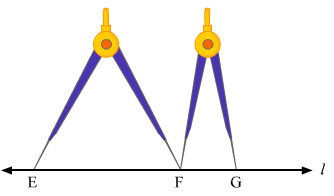(v) Draw a line / and take point Eon it. Now, take a divider and open it such that the ends of both its arms are at A and B. Then, we lift the divider and place its one end at E and other end (say F) on the line 1, as shown in the figure.

Again, lift the divider and place its one end at F and another end (G) on the line 1, opposite to E. Now, reset the divider in such a way that the ends of its one hand are at C and the end of other hand is at D.

Then, we lift the divider and place its one end at G and another end (say H) on the line 1, opposite to E as shown in the figure.

Again, lift the divider and place its one end at H and other end (say I) on the line 1, opposite to E as shown in the figure. Again, lift the divider and place its one end at I and another end (say J) on the line 1, opposite to E as shown in the figure.

EG is required line segment, whose length is equal to (2AB + 3CD).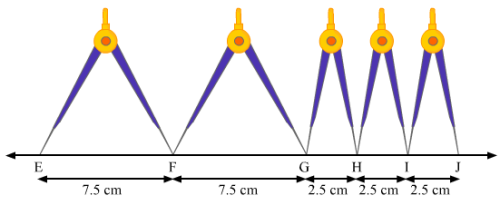Q. 5.) Fill in the blanks:

(i) A part of a line with two end – points is called a Line segment

Explanation: a line segment is a part of a line that is bounded by two distinct end points.

Example: Sides of a triangle or any polygon.

A and B are definite fixed points.

Therefore, AB is a line segment.

(ii)Segment AB is equal to segment BA

Explanation: While naming a line segment, the order may not be same but the length will be equal. If AB = 4 cm, then, BA = 4 cm

(iii) The length of a line segment is the shortest distance between two segments.

Explanation: We measure the length between two points as the length of the line segment between the two points. The length between two points is the straight line, which is the shortest distance between the two end points.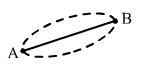(iv) Two segments are congruent only if they have equal length.

(v) Two segments of the same length are said to be congruent.

Explanation: Line segments are congruent if they have the same length. If AB = 4 cm and CD = 4 cm Then, we say segment AB is congruent to segment CD.

Q. 6.) Match the following statements:

 Column A Column B i Line segment has C Two end – point Ii Two segments may intersect A At a point Iii Two segments are congruent B If they have equal lengths iv Line segment is D Portion of a line.

Explanation:

(i) A line segment is a part of a line that is bounded by two distinct end points.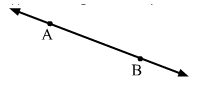(ii) Two line segments will either not intersect at all or intersect at one point. It can never intersect at more than one point.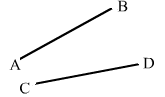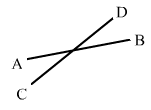(iii) Line segments are congruent if they have the same lengths. If AB = 6 cm and CD = 6 cm Then, AB and CD are congruent.

(iv) A line segment is a part of a line that is bounded by two distinct end points.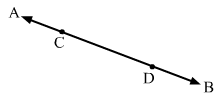Here, AB is a line and CD is a line segment.

So, segment CD is a portion of a line AB.

Q. 7.) Tell which of the following statements are true (T) and false (F):

(i) The intersection of two segments may be segment.

Ans. False

Explanation: Two line segments can intersect maximum at one point and one point cannot make a line segment.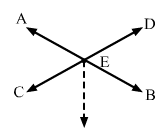(ii) Two segments may intersect at a point which is not any end point of either segments containing it.

Ans. True

Explanation: If two line segment, then point of intersection will not be any of the end points.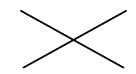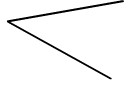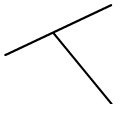(iii) Every ray is a segment

Ans. True

Explanation: A portion of line that starts at point and has no end point is called a ray, whereas align

segment.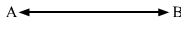(iv) Every segment is a ray.

Both ends points of a line segment are fixed but ray has only one end fixed. Thus a segment can never be

a ray

Q. 8.) What is the difference between a line, a line segment and a ray?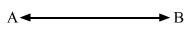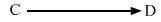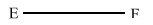A line can be drawn to infinity in both the directions. AB is a line

A line segment has both ends fixed. EF is a line segment. A ray has one end fixed and another end can be drawn to infinity. CD is a ray.

Q. 9.) How many rays are represented in fig 18.8? Name them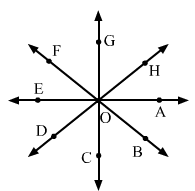We know that a ray has fixed starting point and it can be drawn to infinity. If we take 0 as starting point, we will have a ray in every given direction.

So, our rays are, OA→ , OB→, OC→, OD→, OE→, OF→, OG, OH→ .

Thus, the number of rays in the figure is 8.

103 docs

,

,

,

,

,

,

,

,

,

,

,

,

,

,

,

,

,

,

,

,

,

,

,

,

,

,

,

,

,

,

;